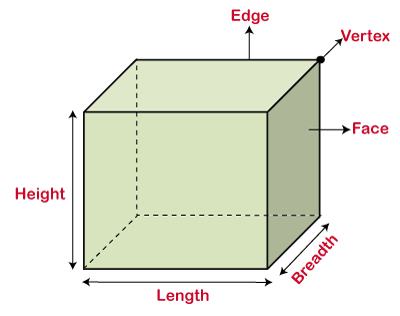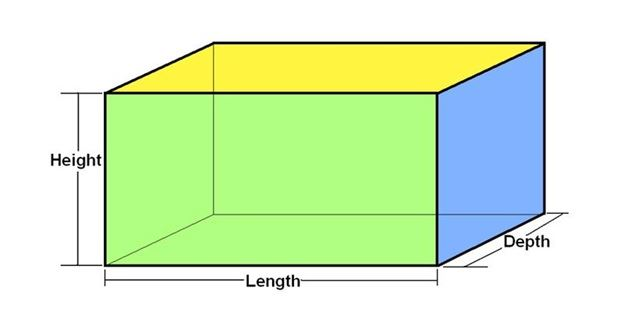Algebraic Identity (a³ - b³)

## Objective

To verify the algebraic identity (a- b3) = (a - b)(a2+ ab + b2)

## Algebraic Identity

• Algebra is a mathematical concept that helps people visualize difficult situations by utilizing mathematical equations.
• It employs variables such as x, y, and z and arithmetic calculations such as addition, elimination, multiplying, and division to produce a coherent mathematical assertion.
• A mathematical and algebraic principle expressed as an equation is an algebraic equation. It’s a two-sided problem on both ends containing formulas.
• The algebraic equation is a simple and rapid approach to solving algebraic problems.
• An algebraic identity is an equality, which is true for all values of the variables in the equality.
• The algebraic equations which are valid for all values of variables in them are called algebraic identities.
• In this way, algebraic identities are used in the computation of algebraic expressions and in solving different polynomials
• Algebraic identities are also used for the factorization of polynomials.

## Pre-requisite Knowledge

###A cube is a three-dimensional shape having all its sides equal and the faces of the cube are square shape.

#### Volume of Cube

• The product of the length of each side itself.

• Formula: Volume = side3

### CuboidA cuboid is also a three-dimensional shape that has three pairs of equal sides parallel to each other and the faces of the cuboid are all rectangular shape.

#### Volume of Cuboid

• The product of its length, breadth, and height

• Formula: Volume = length x breadth x height